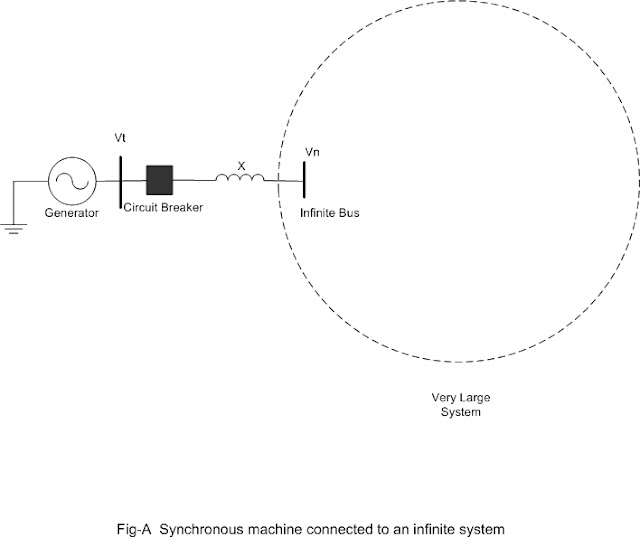### Generator Model and Synchronisation to Infinite Bus

Generator Model

In this article we will discuss about generator model and its synchronization to infinite bus bar. Before doing analysis any machine is required to be properly modeled. The generator model is very important for power system analysis. In general any machine or equipment or device may be modeled in different ways as per the requirement. Here the modelling is for the steady state analysis.

In the diagram we have shown the different ways of representation of generator. Also shown (fig-c) is equivalent circuit (per phase) of generator.

Here the model of synchronous machine is shown as supplying some load in normal state.

Infinite Bus

An infinite bus is one, where injecting power to the grid or drawing power from the grid by the connected machine at the bus, the behivior of the network does not change. See fig-A below. In the diagram the dotted large circle represents the very large electrical power system, operating in normal state. We have shown one bus of this large system where the generator will be synchronized. The step up transformer reactance is represented by X.In the diagram shown consider the generator is of capacity 25 Megawatt. The total capacity of the system is say one lakh Megawatt. Now if the generator will be connected (synchronized) to the grid by closing the breaker, the operating state of the network does not change noticeably from the previous state i.e. before closing the breaker. In other words it is equivalent to say, that the frequency of the network and voltage of the bus Vn does not change.

For synchronising the generator, the following conditions are required to be fulfilled

Requirements for synchronisation

A synchronous generator can only be synchronized to the grid if all the following conditions are fulfilled

• The generator frequency and network frequency are same
• The generator terminal voltage (Vt) and network bus voltage (Vn) are of equal magnitude
• Voltage at the machine terminal (Vt) and network bus voltage (Vn) are in phase
• The generator and the network has the same  phase sequence.
If all the above conditions are satisfied, then the voltage difference across the circuit breaker terminals will be zero and now the breaker is closed. So the machine is now synchronised to the grid.
In a power station control center, synchronizing panels are used for facilitating synchronization work.

For analyzing the behavior of a system, equivalent circuits are required. Below is shown the equivalent circuit diagram for the case of generator synchronized to the grid.

In the actual machine the armature resistance Ra is very small in comparison to the synchronous reactance Xs. So in the equivalent diagram we have neglected Ra. This simplified diagram gives quite accurate result for most practical purposes.

(If you donot like maths, please skip the portion that follows  )

If the power injected to the grid is P, then

P=|E| |Vn| sin(δ) / (Xs+X)
|E|   = Magnitude of no load voltage (phase) of Generator
|Vn| = Magnitude of infinite bus voltage (phase)
δ is the angle between E and Vn phasors
This δ is called power angle.

The above equation, indicates that,

More the value of delta, more will be the power injected to grid.
When delta is more than 90 degrees, the further increase of delta, reduces the power injection to grid.
So delta should be below 90 degrees. Of course, the angle δ  is kept much below 90 degree. Actually δ is desired to be below 30 degrees to give better stability margin to generator.

The above equation is also valid for calculating power flow between two ends of transmission line.
It is concluded that the power that flows from one end to the other end of the line depends on the magnitudes of the voltage waves at two ends, the total series reactance (line resistance ignored being small value)of the line between these two ends and the angle between the two end voltage phasors.

Note: In our above discussion, the voltage and current means rms values not the maximum value. The nominal voltage standards like 33kV and 220 kV etc are rms line voltage values. The per phase voltage is found by dividing line voltage by 1.73.( or, Vph= V/ √3). (Vl is the line voltage)

We have studied some important behavior of synchronous generator in relation to the grid. Some other properties we will discuss later.
You might be eager to know about transformer. I discuss some basics about the transformer in next post.